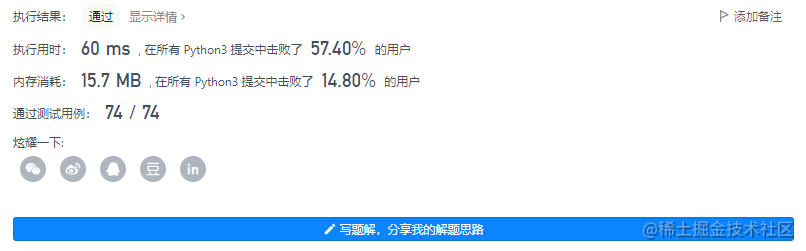# leetcode_845 陣列中的最長山脈

### 要求

• B.length >= 3
• 存在 0 < i < B.length - 1 使得 B < B < ... B[i-1] < B[i] > B[i+1] > ... > B[B.length - 1] （注意：B 可以是 A 的任意子陣列，包括整個陣列 A。）

### 核心程式碼

```python class Solution: def longestMountain(self, arr: List[int]) -> int: if len(arr) < 3: return 0

``````    def is_peak(index):
return 1 <= index <=len(arr) - 2 and arr[index - 1] < arr[index] and arr[index] > arr[index + 1]

def find_all_peaks():
return [index for index in range(len(arr)) if is_peak(index)]

def get_len_of_the_moutain(index):
left = right = index
length = 1
while left > 0 and arr[left - 1] < arr[left]:
left -= 1
length += 1
while right < len(arr) - 1 and arr[right] > arr[right + 1]:
right += 1
length += 1
return length

return max([get_len_of_the_moutain(peak) for peak in find_all_peaks()] + )
``````

```